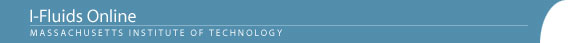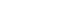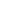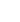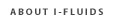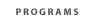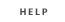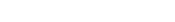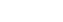2.25: Advanced Fluid Dynamics Section 1: Continuum viewpoint and the equation of motion Section 2: Static Fluids Section 3: Mass Conservation Section 4: Inviscid flow - differential approach Section 5: Control Volume Theorums Section 6: Navier-Stokes equation and viscous flow Section 7: Similarity and dimensional analysis Section 8: Boundary layers, separation and effect on drag/lift Section 9: Vorticity and circulation Section 10: Potential flows; lift, drag and thrust production Section 11: Surface tension and its effect on flows Section 12: Introduction to turbulence Back to 2.25 HomeSection 1: The Continuum Viewpoint and the Equation of Motion1.1 On the molecular origin of fluid behavior and its analytical difficulties.
1.2 The continuum viewpoint and the concept of properties as fields; Eulerian vs Lagrangian methods of description. The importance of reference frame in the Eulerian description; steady and unsteady flows.
1.3 Material particles, material volumes, and control volumes. The physical laws that govern the behavior of continua, expressed in terms of material volumes.
1.4 Forces acting on a continuum: body forces and surface forces.
1.5 Rheological properties of materials; elastic solids, Newtonian fluids and viscosity, non-Newtonian fluids, viscoelastic fluids. The defining attribute of a "fluid."
1.6 Pascal's principles and the concept of pressure in a static (or otherwise shear-free) fluid.
1.7 Thermal equation of state for fluids of constant composition (the equilibrium density-pressure-temperature relation).
1.8 Net surface force on a fluid particle in a pressure field.
1.9 Acceleration of a fluid particle expressed in terms of the (Eulerian) velocity field: the material (or substantial, or total) time derivative. The rate of change of with time of a fluid property seen by an observer moving through a continuum at an arbitrary velocity.
1.10 The equation of motion of a fluid in differential form.
Reading
Fay, Chapter 1, Chapter 2: pages 44-75, Chapter 3: pages 89-97, Chapter 4: pages 128-132

Problem Set Chapter - 1

Handout: Special Problem 1.1 on sounding rocket